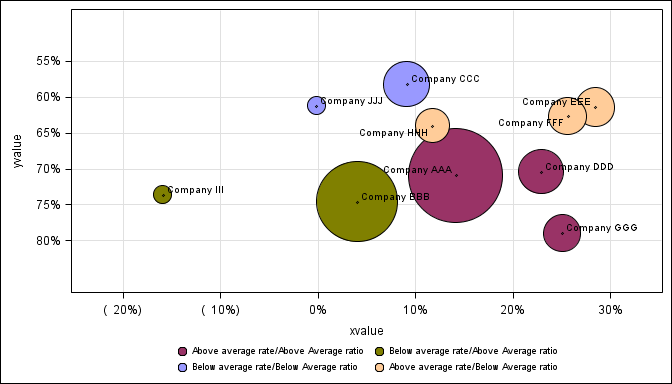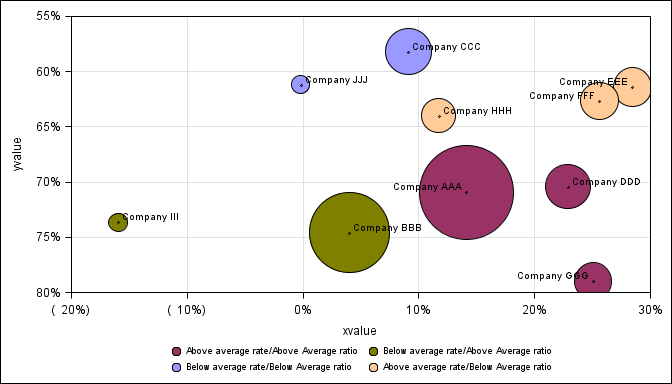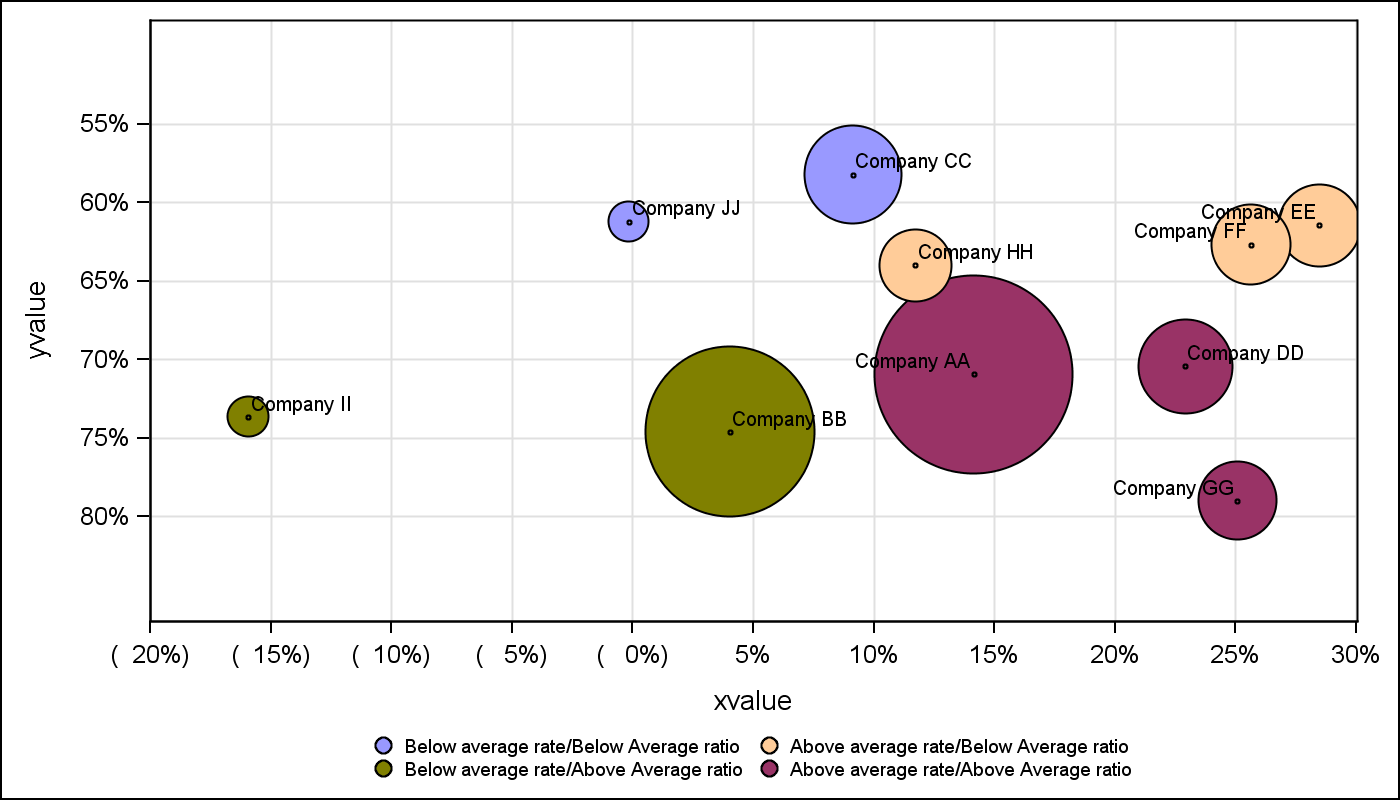## sgplot bubble xaxis values and legend order

Hello, I am using SAS 9.3.  (1) Is there a way to add more tick values to the xaxis? In this particular example, how can I add (15%), (5%), 5%, 15%, 25% to the existing xaxis?  This graph code is inside a macro and is used to create many graphs (For many states and many products in each state) so the data varies for each state (this example is only for one state, XX), therefore there can be no hard coded values in a VALUES=  statement.

(2) Is there a way to force the legend to always be in a static order?  For this particular example I would like the legend to always be in the order shown belowa no matter what the size of the bubble is:

Below average rate/Below Average ratio           Above average rate/Below Average ratio

Below average rate/Above Average ratio          Above average rate/Above Average ratio

Below is my code and an image of the bubble chart:

data graphdataone;

infile datalines;

input

state   \$ 1-2

product \$ 4-7

rank    \$ 9-10

name    \$ 12-22

sizeamt 24-32

xvalue  36-43

yvalue  45-51

;

datalines;

XX Auto 1   Company AAA   776725.00   0.14153    0.7096    Above average rate/Above Average ratio

XX Auto 2   Company BBB   583933.00   0.04045    0.74602   Below average rate/Above Average ratio

XX Auto 3   Company CCC   225388.00   0.09141    0.58228   Below average rate/Below Average ratio

XX Auto 4   Company DDD   213436.00   0.22914    0.70447   Above average rate/Above Average ratio

XX Auto 5   Company EEE   174198.00   0.28477    0.61449   Above average rate/Below Average ratio

XX Auto 6   Company FFF   165755.00   0.25635     0.62687   Above average rate/Below Average ratio

XX Auto 7   Company GGG  163169.00   0.25077    0.78992   Above average rate/Above Average ratio

XX Auto 8   Company HHH   147465.00   0.11738    0.64021   Above average rate/Below Average ratio

XX Auto 9   Company III        78837.00    -0.15926   0.73657   Below average rate/Above Average ratio

XX Auto 10 Company JJJ      78170.00    -0.00147   0.61234   Below average rate/Below Average ratio

;

ods graphics on / reset

imagename = 'TestBubble'

outputfmt=png

antialias=on

height = 4in

width = 7in

scale=on;

data attrmap;

id='A'; value='Below average rate/Below Average ratio'; fillcolor='CX9999FF'; textcolor='black'; output;

id='A'; value='Above average rate/Below Average ratio'; fillcolor='CXFFCC99'; textcolor='black'; output;

id='A'; value='Below average rate/Above Average ratio'; fillcolor='CX808000'; textcolor='black'; output;

id='A'; value='Above average rate/Above Average ratio'; fillcolor='CX993366'; textcolor='black'; output;

run;

title;

footnote;

proc sgplot data = work.graphdataone noautolegend dattrmap=attrmap;

bubble  x = xvalue y = yvalue size = sizeamt / group=quad

attrid=A

LINEATTRS=(Color= "black")

name='b1';

scatter x = xvalue y = yvalue / datalabel=name

markerattrs=(size=3);

yaxis grid

reverse

TICKVALUEFORMAT=percent7.0

thresholdmax=1

thresholdmin=1;

xaxis grid

TICKVALUEFORMAT=percent7.0

thresholdmax=1

thresholdmin=1;

keylegend 'b1' / valueattrs = (size=7) across=2 noborder;

run;(3) I would like the xaxis and yaxis to begin and end on a tick mark.  I have tried offsetmin=0, offsetmax=0, thresholdmin=1 and thresholdmax=1 on both the xaxsis and yaxis. On some of the graphs, depending on the size of the bubbles, some of the bubbles get chopped off.  Below Company EEE and Company GGG get chopped.Jay54
Meteorite | Level 14

## Re: sgplot bubble xaxis values and legend order

1.  Yes, you can supply the values=(-0.2 to 0.3 by 0.05) on the x axis to get the tick values you want.  This will also set the axis data min and max to the extreme values you provide.  However, some values may not display if there is not enough space on the axis, and they collide.  To see the values you provide either make the width of graph bigger, reduce the font size or set appropriate FitPolicy.

2.  The legends are in the order in which the group values are encountered in the data.  First group value in data is first in legend.  To force the legend to always be in the order you want, you can add dummy values at the top of the data.with the appropriate group values but missing x or y axis value.

3.  Axis tick values start drawing based on THRESHOLD.  To always get an outer tick mark, set axis THRESHOLDMIN and MAX to 1. Refer doc for explanation of THRESHOLD.  In your case you are already using Threshold min and max.  Since you are adding scatter for data labels, the data label is setting offsets.  you can set offsetmin and max = 0.  Note, bubble at upper end of x axis is clipped.Discussion stats